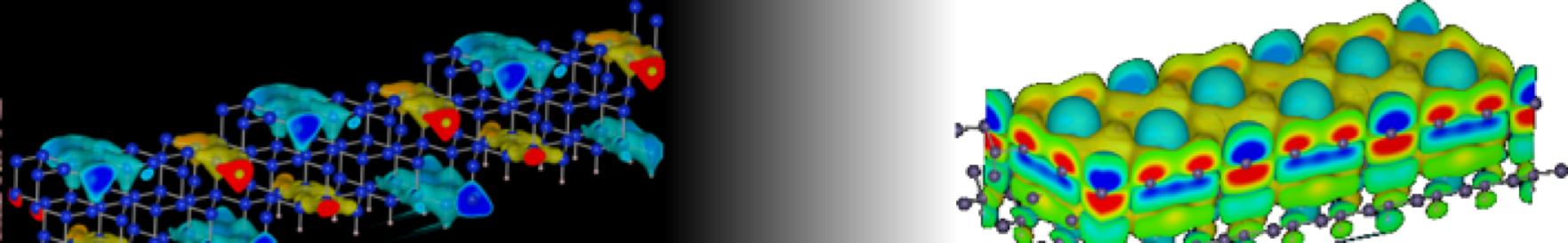SST Lab Dokuwiki Header# 電子物性特論

キーワード
Hartree-Fock theory,
Density functional theory,
Pseudo-potential,
Atomic-scale simulation environment

## 講義目的

It is the objective of the theory for nano-electronic devices to understand the fundamental principles that determine the atomic arrangement and the electronic structure of the nano-devices and the processes occurring in the nano-devices. The recent microscopic theoretical treatment allows to describe various properties of the nano-devices from first principles. In this class, students study the concept of the first principles calculations, the approximation methods which are commonly used and their limitation, and the practical implementation of a density functional code.

## 達成目標

Students can

1. explain the basic concept and outline of the first principles calculations,
2. execute the calculation for a small molecule for example a carbon monoxide, and
3. perform the critical reading of the manuscripts concerned about the first principles calculations.

## 成績評価

Contribution for reading the text (40 percent of the grade) and Practice reports (60 percent of the grade) for objectives of this course.

## 授業内容

9. Reading manuscript about and carrying out basic practice on Atomic-scale simulation environment
10. Carrying out Practical work for total energy calculation
11. Carrying out Practical work for Hellmann-Feynman force
12. Carrying out Practical work for charge density and wave functions
13. Carrying out Practical work for density of state
14. Carrying out Practical work for electronic band dispersion
15. Carrying out Practical work for visualisation of calculation results

## 準備学習

1. Students need to summarise the contents of the solid state physics in undergraduate classes.
2. Students need to read the part about Hartree-Fock theory in the textbook.
3. Students need to read the part about Quantum chemistry method in the textbook.
4. Students need to read the part about Density functional theory in the textbook.
5. Students need to read the part about Pseudopotentials in the textbook.
6. Students need to read the part about Implementations of density functional theory in the textbook.
7. Students need to read the part about Further many-electron method in the textbook.
8. Students need to read the part about Tight-binding model in the textbook.
9. Students need to read documents about Atomic-scale simulation environment on the Web.
10. Students need to prepare the code to calculate the total energy of a small molecule.
11. Students need to finish the report about total energy calculation and to prepare the code to calculate Hellmann-Feynman forces for a small molecule.
12. Students need to finish the report about Hellmann-Feynman force and to be familiar with VESTA code.
13. Students need to finish the report about charge density and wave functions and to prepare the code to calculate a small molecule and a simple metal crystal with various K-point sampling.
14. Students need to finish the report about density of state and to prepare the code to calculate a simple metal crystal with K-point sampling on some symmetric lines in the first Brillouin zone.
15. Students need to finish the report about electronic band dispersion, to prepare the code to optimise the structure of a small molecule and to be familiar with Jmol code.

## 教科書・参考書

• Theoretical Surface Science —- Microscopic Perspective —- A. Groß, Springer-Verlag, 2003. ISBN 3-540-43903

Copies of Manuscripts are provided. (No need to buy the textbook)

## 備考

• Participants have to give a presentation about assigned parts of the manuscripts. Practical works are carried out at the Laboratory in Bld.C5-3F.
• This class is managed with the seminar style.
• Feedback for practical works is given as occasion demands in the class